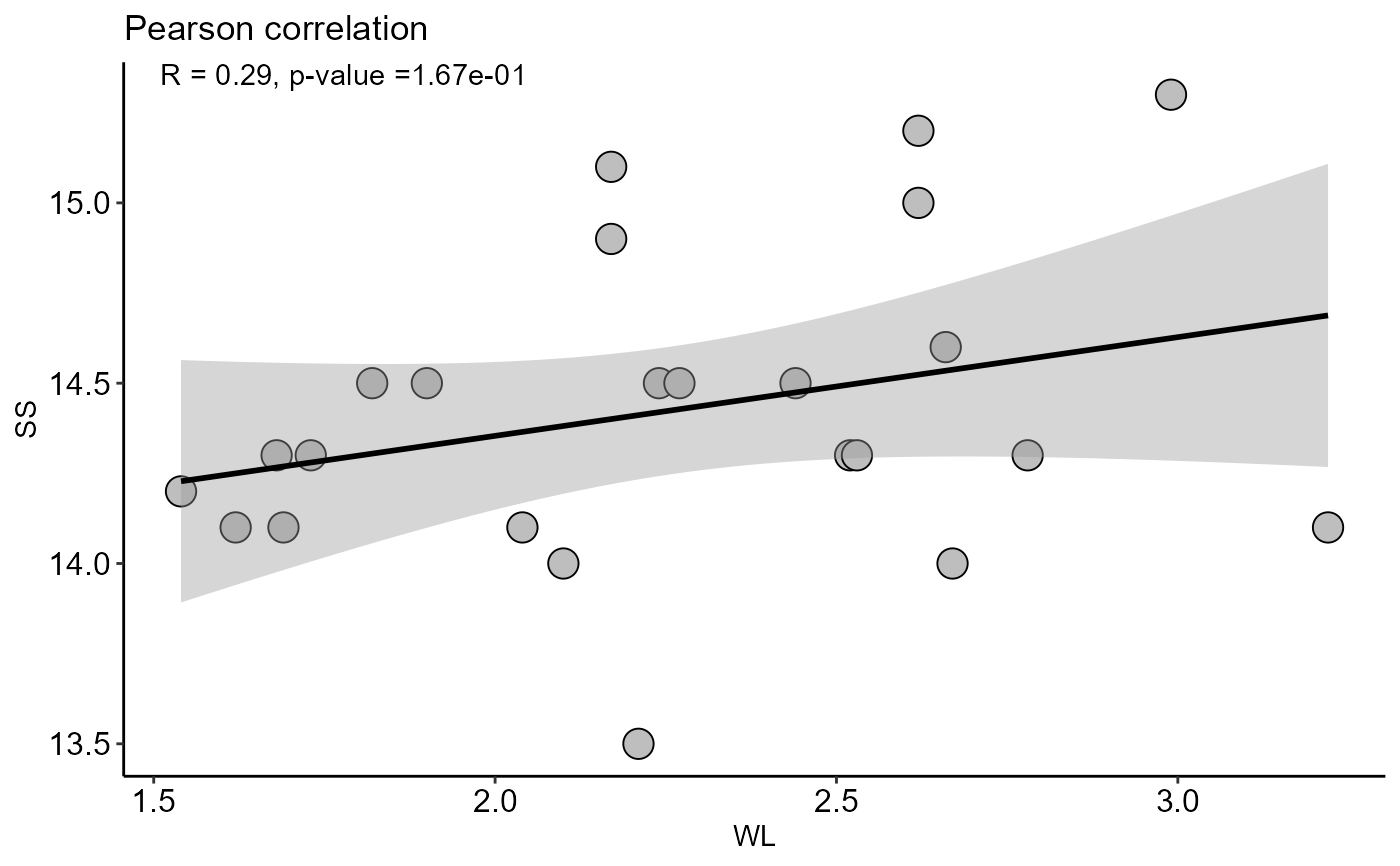Correlation analysis function (Pearson or Spearman)

plot_cor(
x,
y,
method = "pearson",
ylab = "Dependent",
xlab = "Independent",
theme = theme_classic(),
pointsize = 5,
shape = 21,
fill = "gray",
color = "black",
axis.size = 12,
ic = TRUE,
title = NA,
family = "sans"
)

## Arguments

x

Numeric vector with independent variable

y

Numeric vector with dependent variable

method

Method correlation (default is Pearson)

ylab

Variable response name (Accepts the expression() function)

xlab

Treatments name (Accepts the expression() function)

theme

ggplot2 theme (default is theme_classic())

pointsize

Point size

shape

shape format

fill

Fill point

color

Color point

axis.size

Axis text size

ic

title

title

family

Font family

## Value

The function returns a graph for correlation

## Author

Gabriel Danilo Shimizu, shimizu@uel.br

Leandro Simoes Azeredo Goncalves

Rodrigo Yudi Palhaci Marubayashi

## Examples

data("pomegranate")
with(pomegranate, plot_cor(WL, SS, xlab="WL", ylab="SS"))
#> geom_smooth() using formula = 'y ~ x'